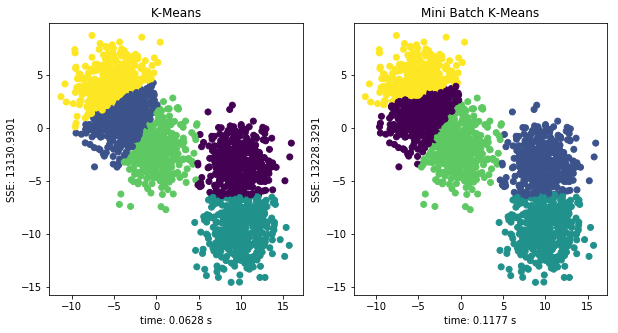# 机器学习|划分聚类之 K-Means 详解 (Python 语言描述)

## K-Means 聚类方法

 对于未聚类数据集，首先随机初始化 K 个（代表拟聚类簇个数）中心点，如图红色五角星所示。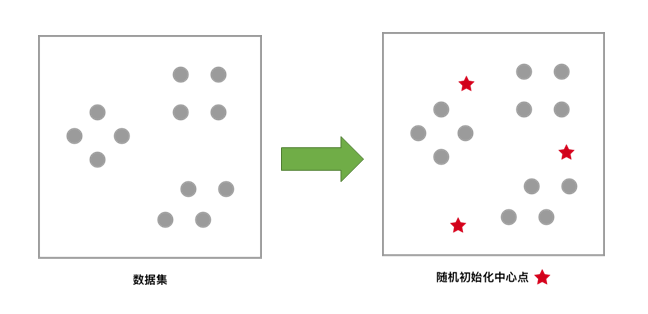每一个样本按照距离自身最近的中心点进行聚类，等效于通过两中心点连线的中垂线划分区域。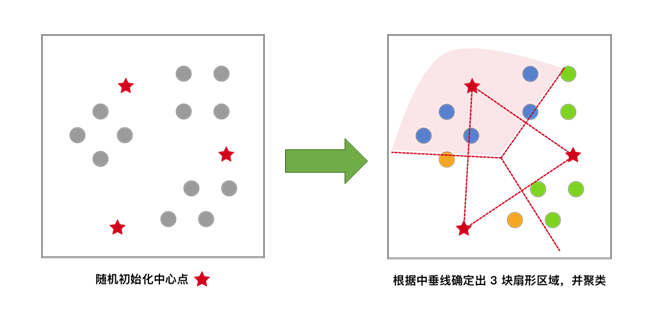依据上次聚类结果，移动中心点到个簇的质心位置，并将此质心作为新的中心点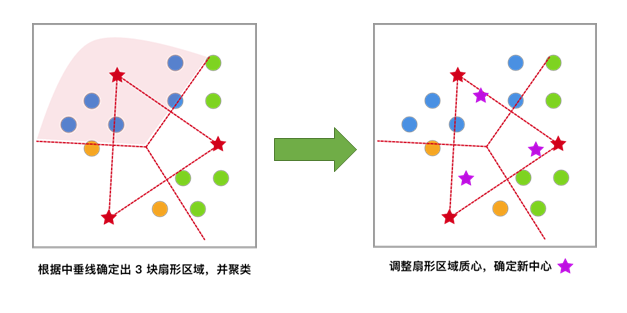反复迭代，直至中心点的变化满足收敛条件（变化很小或几乎不变化），最终得到聚类结果。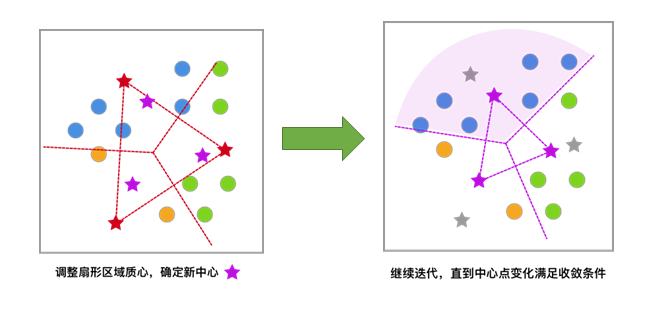### 生成示例数据

• n_samples：表示生成数据总个数,默认为 100 个。
• n_features：表示每一个样本的特征个数，默认为 2 个。
• centers：表示中心点的个数，默认为 3 个。
• center_box：表示每一个中心的边界,默认为 -10.0到10.0。
• random_state：表示生成数据的随机数种子。

• data：表示数据信息。
• label：表示数据类别。

array([[ 8.28390539,  4.98011149],
[ 7.05638504,  7.00948082],
[ 7.43101466, -6.56941148],
[ 8.20192526, -6.4442691 ],
[ 3.15614247,  0.46193832],
[ 7.7037692 ,  6.14317389],
[ 5.62705611, -0.35067953],
[ 7.53828533, -4.86595492],
[ 8.649291  ,  3.98488194],
[ 7.91651636,  4.54935348]])


### 数据可视化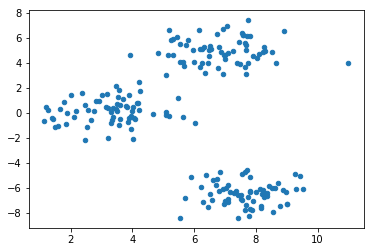### 随机初始化中心点

array([[ 6.42802708, -1.51776689],
[ 3.09537831,  1.97999275],
[ 1.11702824, -0.27169709]])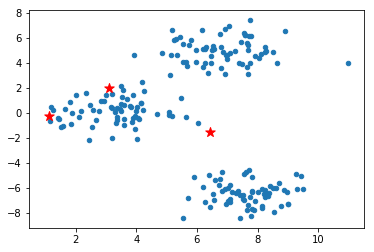### 计算样本与中心点的距离

$$d_{euc}= \sqrt{\sum_{i=1}^{N}(X_{i}-Y_{i})^{2}} \tag{1}$$

• X, Y ：两个数据点
• N：每个数据中有 N 个特征值，
• X_{i} ：数据 X 的第 i 个特征值

### 最小化 SSE，更新聚类中心

$$SSE(C)=\sum_{k=1}^{K}\sum_{x_{i}\in C_{k}}\left | x_{i}-c_{k} \right |^{2} \tag{2}$$

$$c_{k}=\frac{\sum_{x_{i} \in C_{k}}x_{i}}{I(C_{k})} \tag{3}$$

$$I(C_{k})$$ 表示在第 k 个集合 $$C_{k}$$ 中数据的个数。

### K-Means 聚类算法实现

K-Means 算法则采用的是迭代算法，避开优化 SSE 函数，通过不断移动中心点的距离，最终达到聚类的效果。

#### 算法流程

1. 初始化中心点：判断数据集可能被分为 k 个子集，随机生成 k 个随机点作为每一个子集的中心点。
2. 距离计算，类别标记：样本和每一个中心点进行距离计算，将距离最近的中心点所代表的类别标记为该样本的类别。
3. 中心点位置更新：计算每一个类别中的所有样本的均值，作为新的中心点位置。
4. 重复 2，3 步骤，直到中心点位置不再变化。

#### 算法实现

array([[ 7.67007252, -6.44697348],
[ 6.83832746,  4.98604668],
[ 3.28477676,  0.15456871]])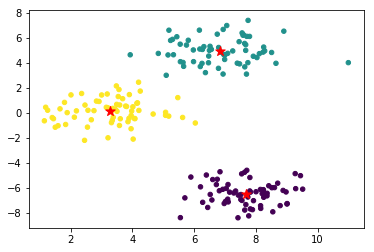### 中心点移动过程可视化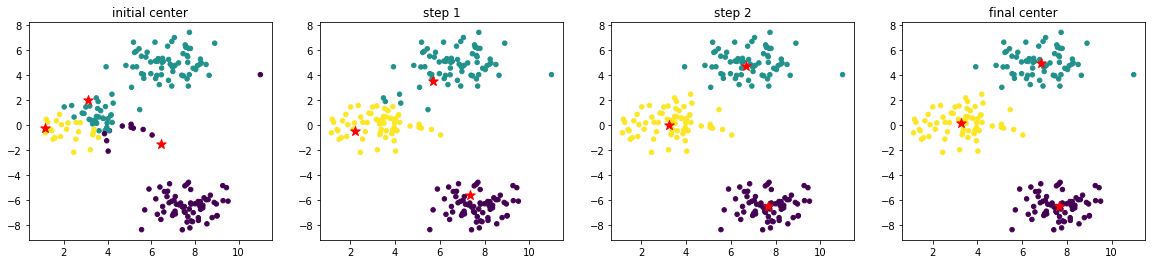• 初始化中心点的位置很好，比较均匀分布在了数据范围中。如果初始化中心点集中分布在某一角落，迭代次数肯定会增加。
• 示例数据分布规整和简单，使得无需迭代多次就能收敛。

### K-Means 算法聚类中的 K 值选择

• X：表示需要聚类的数据。
• n_clusters：表示聚类的个数，也就是 K 值。

• centroid：表示中心点坐标。
• label：表示聚类后每一个样本的类别。
• inertia：每一个样本与最近中心点距离的平方和，即 SSE。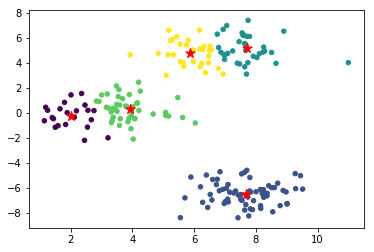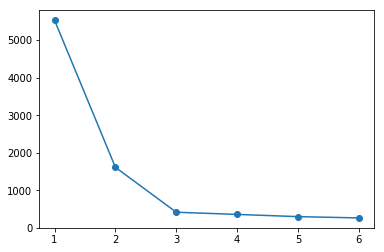## K-Means++ 聚类算法

### 生成示例数据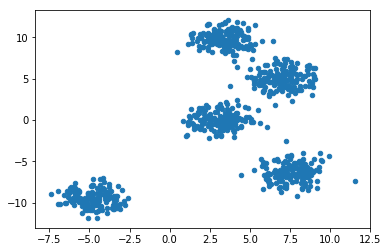### 随机初始化中心点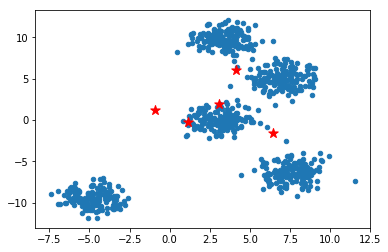### K-Means 聚类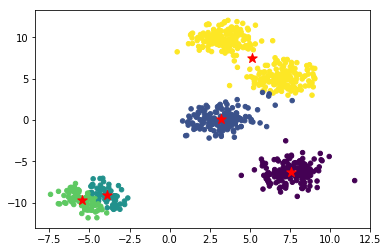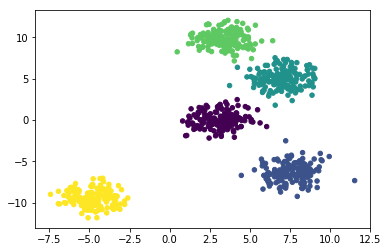### K-Means++ 算法流程

K-Means++ 相较于 K-Means 在初始化中心点上做了改进，在其他方面和 K-Means 相同。

1. 在数据集中随机选择一个样本点作为第一个初始化的聚类中心。
2. 计算样本中的非中心点与最近中心点之间的距离 D(x) 并保存于一个数组里，将数组中的这些距离加起来得到 $$Sum(D(x))$$。
3. 取一个落在 $$Sum(D(x))$$范围中的随机值 R ，重复计算 $$R=R-D(x)$$ 直至得到 $$R\leq0$$ ，选取此时的点作为下一个中心点。
4. 重复 2,3 步骤，直到 K 个聚类中心都被确定。
5. 对 K 个初始化的聚类中心，利用 K-Means 算法计算最终的聚类中心。

### K-Means++ 算法实现

K-Means++ 在初始化样本点之后，计算其他样本与其最近的中心点距离之和，以备下一个中心点的选择，下面用 Python 来进行实现：

array([[ 4.1661903 ,  0.81807492],
[ 8.9161603 ,  5.58757202],
[ 7.62699601,  2.3492678 ],
[-3.42049424, -9.57117787],
[ 3.35681598, -0.54000802]])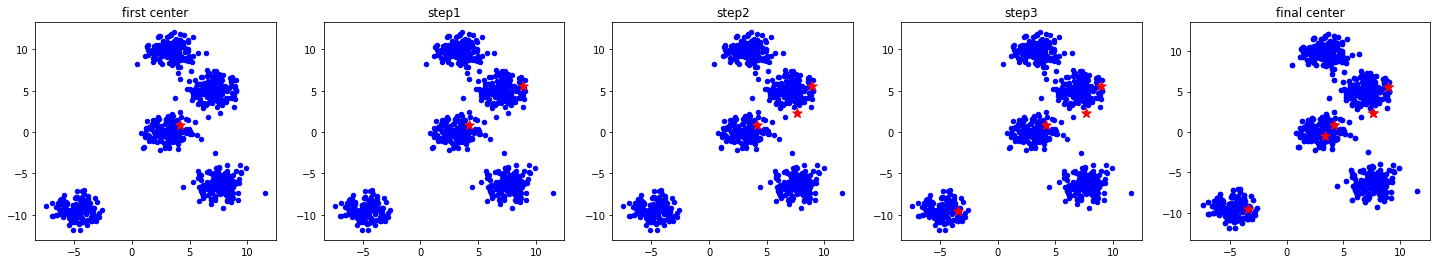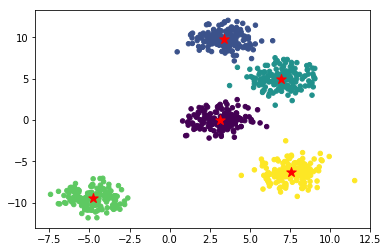## Mini-Batch K-Means 聚类算法